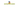# How to loop through an array in JavaScriptIn this tutorial, we are going to learn about different ways to loop through an array in JavaScript.

## For loop

`for loop` helps us to loop through an array and access the elements inside an array.

Example:

``````const arr = [1,2,3,4];

for (let i=0;i<arr.length;i=i+1){
console.log(arr[i]);
}

//output
// first iteration  1
//second iteration 2
//third iteration 3
//fourth iteration 4``````

## For in loop

`for in` loop gives us `indexes` of the array by using that `indexes` we can access the array elements.

Example:

``````for (let index in arr){
console.log(arr[index]);
}``````

## For of loop

`for..of` loop directly gives us `elements` of the array instead of `indexes`.

Example:

``````for(let element of arr){
console.log(element);
}``````

## forEach method

By using `forEach()` method, we can also access the array elements directly.

``arr.forEach(element=>console.log(element));``

The `forEach` method runs the provided callback function once on each element present in the array.

## While loop

``````let index= 0; //initialized with 0

while(index < arr.length){
console.log(arr[index]); //logging element
index = index+1; //incrementing the index
}``````